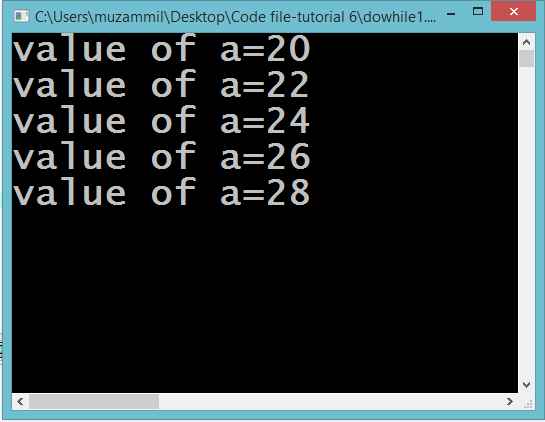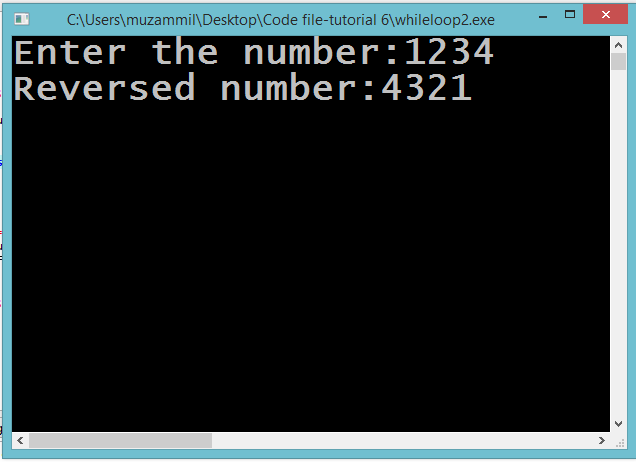# Do While Loops

## Do While loops

In this part you will learn: 1. C syntax 2. Do While loops In this tutorial I will teach you about Do While loops. We will see a very basic program at first to grasp the concept of while loop and then afterwards we will make another program of reversing a number. Do While Loop Basic Step: Open Dev C++ then File > new > source file and start writing the code below.
`#include<stdio.h>#include<conio.h>`
These two are the most common and basic header files. The first one stdio.h is used to grant us most of the basic functions of C like Input and Output functions. The second one conio.h provides us functions like getch() which is used to pause our screen until we press a button.
`int main(){	int a=20; 	do	{		printf("value of a=%d\n",a); 		a=a+2; 	}while(a<30); 	getch();  }`
This is a very simple program. We will see a very basic do while loop in this program. The do while loop is almost similar to the previous loop i.e for loop and while loop. The for loop had three statements which were necessary for a for loop to work. The while loop had a single compulsory statement which was the condition that runs the while loop. Now similarly the do while loop also has the condition statement. This condition statement is necessary for the do while loop to run. The difference between the do while loop and the while loop is that there can be a possibility that a while loop might be present in the program but may not run at least once depending upon the condition of the loop. But a do while loop despite of the condition runs at least once. It is not possible for do while loop to be present in the program and not even run for a single time. Coming toward the program. We initialized a integer variable at the start and then we enter the loop. We printed its value and then added 2 in the integer variable. Then after the closing brackets of the body of the loop the condition is tested for the first time. If it is true the loops executes again otherwise the program breaks out of the loop. Execute > compile then Execute > run OutputReversing a Number Basic Step: Open Dev C++ then File > new > source file and start writing the code below.
`#include<stdio.h>#include<conio.h>`
These two are the most common and basic header files. The first one stdio.h is used to grant us most of the basic functions of C like Input and Output functions. The second one conio.h provides us functions like getch() which is used to pause our screen until we press a button.
`int main(){	int num,digit;	printf("Enter the number:");	scanf("%d",&num);  	printf("Reversed number:");	do	{		if(num>0)		{			digit=num%10;			num=num/10;			printf("%d",digit); 		}	}while(num>0);  	getch(); }`
In this program are reversing a number that will be entered by the user using a do while loop. At the start we took the input from the user in a integer variable ‘num’. Then we entered the body of the loop the first time. In there we stored the remainder of the number in an other variable digit by dividing it by 10. Then we divided the number by 10 and saved it back in the ‘num’ variable. This way we separated the last digit of the number for it. Then afterwards there is the condition that till the num is greater than 0 the loop should keep on running. Then outside the loop there is only the getch() function for the program to pause. Execute > compile then Execute > run Output